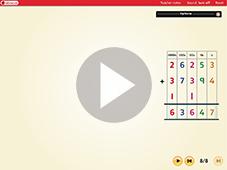Compact addition Worksheet Answers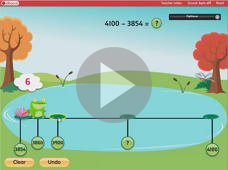Counting up Worksheet Answers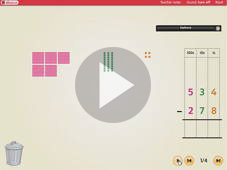Compact subtraction Worksheet AnswersLadder multiplication Worksheet Answers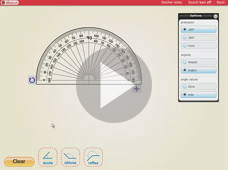Using a protractor to measure angles Worksheet Answers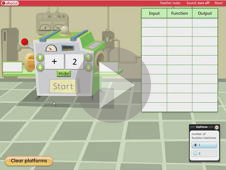Function machines Worksheet Answers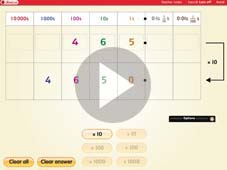Multiplying and dividing by 10, 100 and 1000 Worksheet Answers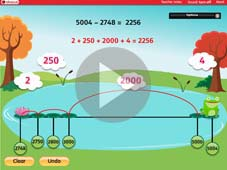Counting up to find a difference Worksheet Answers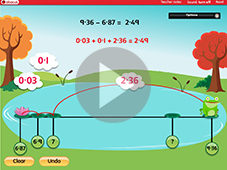Counting up to find a decimal difference Worksheet Answers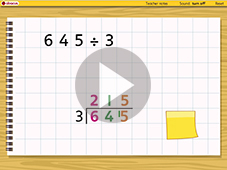Use short division Worksheet Answers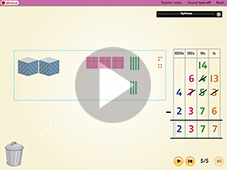Subtracting 4 digit numbers Worksheet AnswersAdding 5 digit numbers Worksheet Answers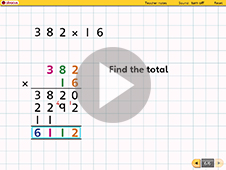Multiplying 3 digit by teen numbers Worksheet Answers